## RS Aggarwal Class 9 Solutions Chapter 7 Areas Ex 7A

These Solutions are part of RS Aggarwal Solutions Class 9. Here we have given RS Aggarwal Class 9 Solutions Chapter 7 Areas Ex 7A.

Question 1.
Solution:
Base of the triangle (b) = 24cm and height (h) = 14.5 cm
∴ Area = $$\frac { 1 }{ 2 }$$ x b x h = $$\frac { 1 }{ 2 }$$ x 24 x 14.5 cm²
= 174 cm² Ans.

Question 2.
Solution:
Let the length of altitude of the triangular field = x then its base = 3x.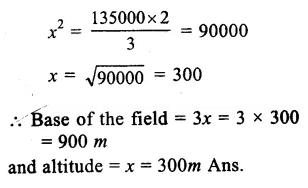Question 3.
Solution:
Sides of a triangle = 42cm, 34cm and 20cm
Let a = 42cm, b = 34cm and c = 20 cm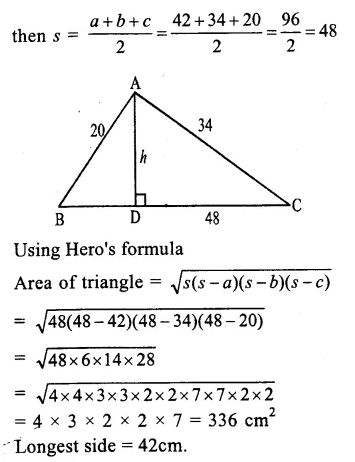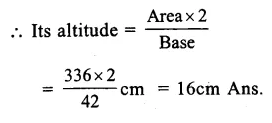Question 4.
Solution:
Sides of the triangle = 18cm, 24cm and 30cm
Let a = 18 cm, b = 24 cm and c = 30cm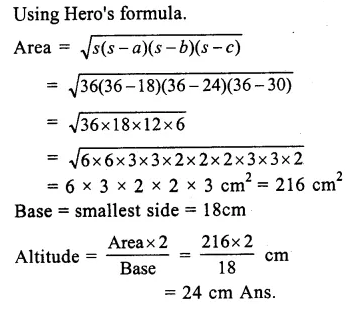Question 5.
Solution:
Sides of triangular field ABC arc 91m, 98m and 105m
Let AC be the longest side
∴ BD⊥AC
Here a = 98m, b = 105m and c = 91m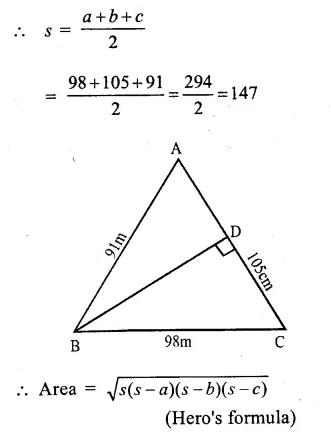Question 6.
Solution:
Perimeter of triangle = 150m
Ratio in the sides = 5:12:13
Let sides be 5x, 12x and 13x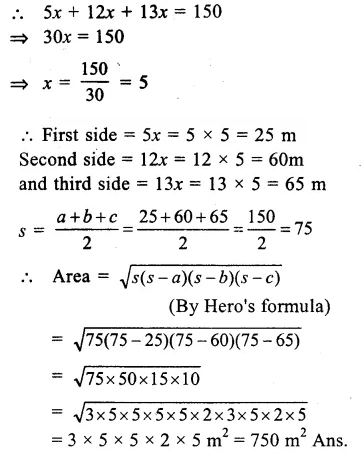Question 7.
Solution:
Perimeter of a triangular field = 540m
Ratio is its sides = 25 : 17 : 12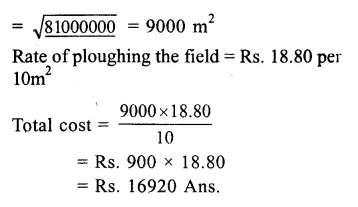Question 8.
Solution:
Perimeter of the triangular field = 324 m
Length of the sides are 85m and 154m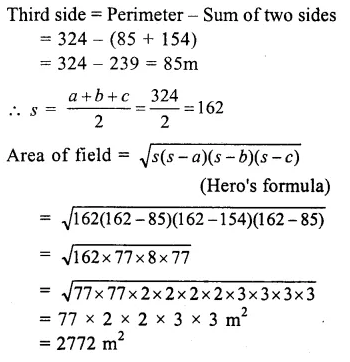Question 9.
Solution:
Length of sides are
13 cm, 13 cm and 20cm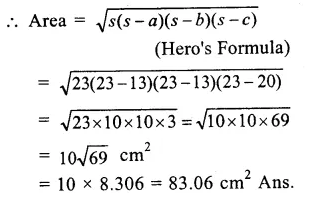Question 10.
Solution:
Base of the isosceles triangle ABC = 80cm
Area = 360 cm²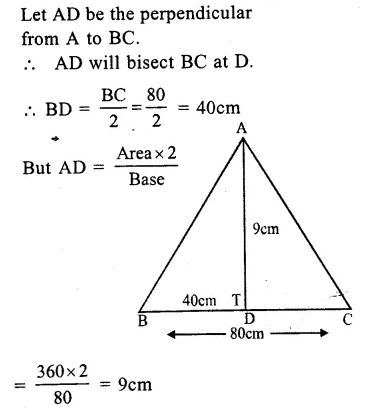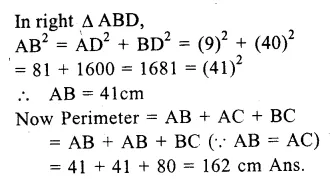Question 11.
Solution:
Perimeter of the triangle
ABC = 42 cm.
Let length of each equal sides = x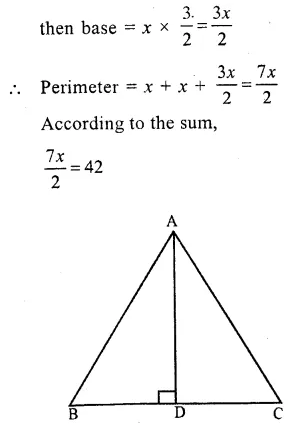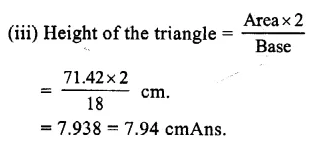Question 12.
Solution:
Area of equilateral triangle = 36√3 cm².
Let length of each side = aQuestion 13.
Solution:
Area of equilateral triangle = 81√3 cm²
Let length of each side = a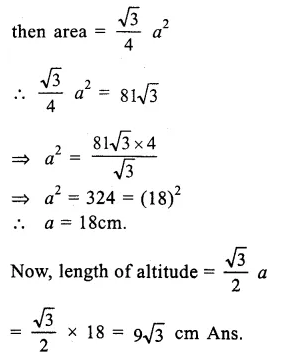Question 14.
Solution:
∆ ABC is a right angled triangle, right angle at B.
∴ BC 48cm and AC = 50cm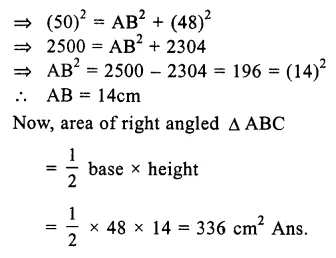Question 15.
Solution:
Each side of equilateral triangle
(a) = 8cm.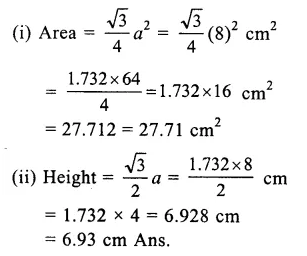Question 16.
Solution:
Let a be the each side of
the equilateral triangle.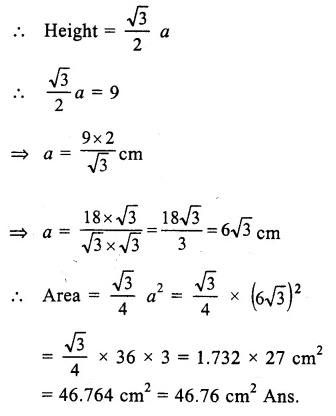Question 17.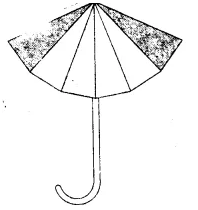Solution:
The given umbrella has 12 triangular pieces of the size 50cm x 20cm x 50cm. We see that each piece is of an isosceles triangle shape and we have to find firstly area of one such triangle.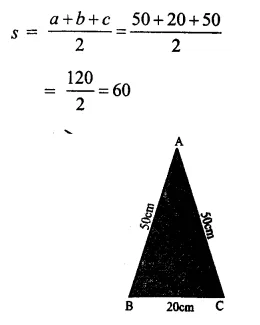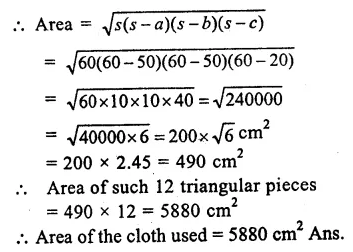Question 18.
Solution:
The given floral design is made of 16 tiles
The size of each tile is 16cm 12cm, 20cm
Now we have to find the area of firstly one tile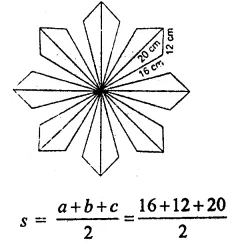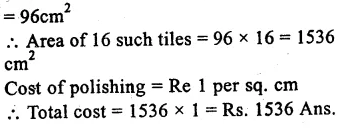Question 19.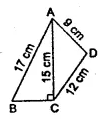Solution: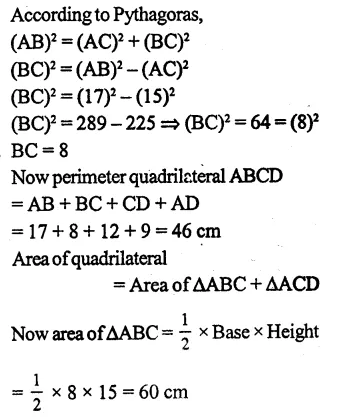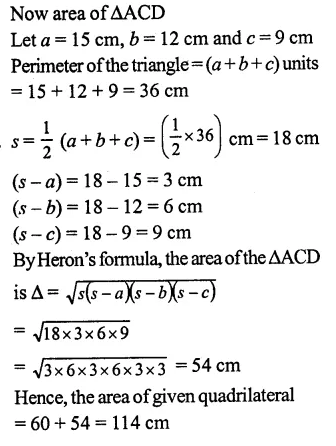Question 20.
Solution:
In the figure, ABCD is a quadrilateral
AB = 42 cm, BC = 21 cm, CD = 29 cm,
DA = 34 cm and ∠CBD = 90°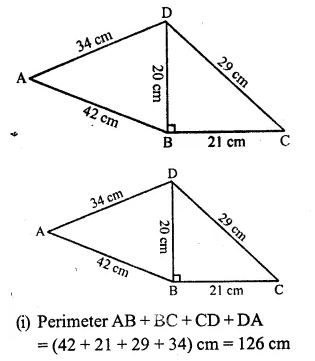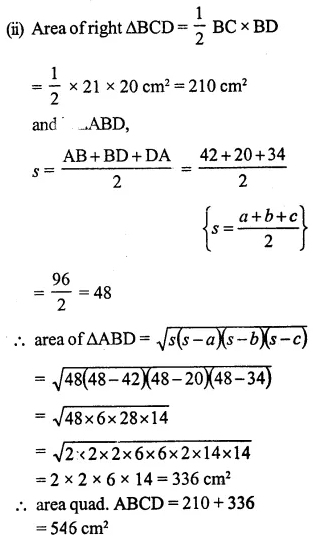Question 21.
Solution:
from the figure
∆DAB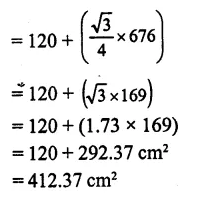Question 22.
Solution: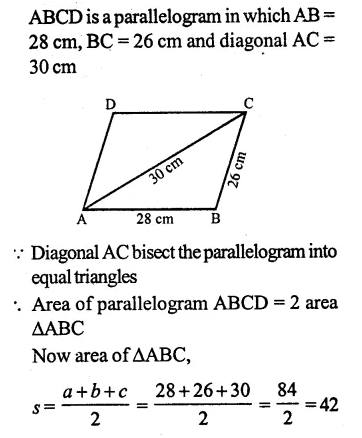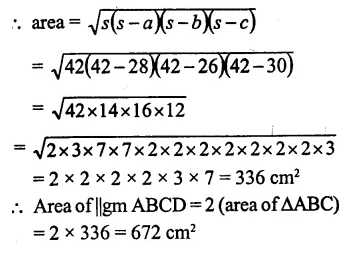Question 23.
Solution:
from the figure,
We know that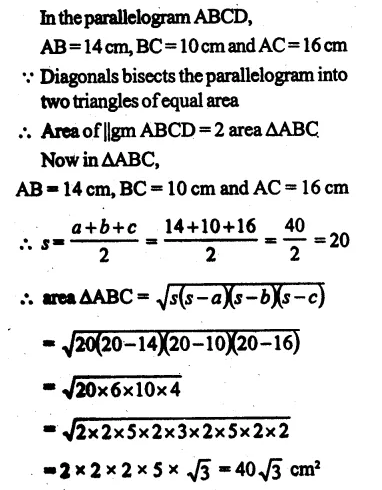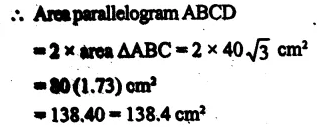Question 24.
Solution: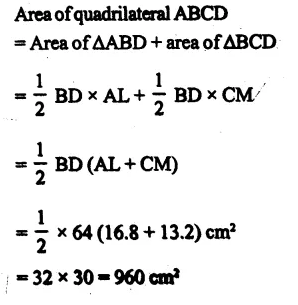Hope given RS Aggarwal Class 9 Solutions Chapter 7 Areas Ex 7A are helpful to complete your math homework.

If you have any doubts, please comment below. Learn Insta try to provide online math tutoring for you.